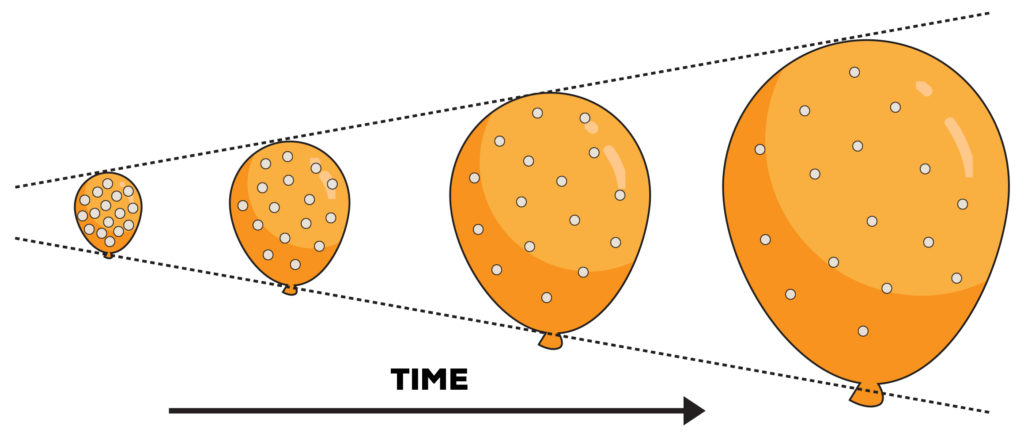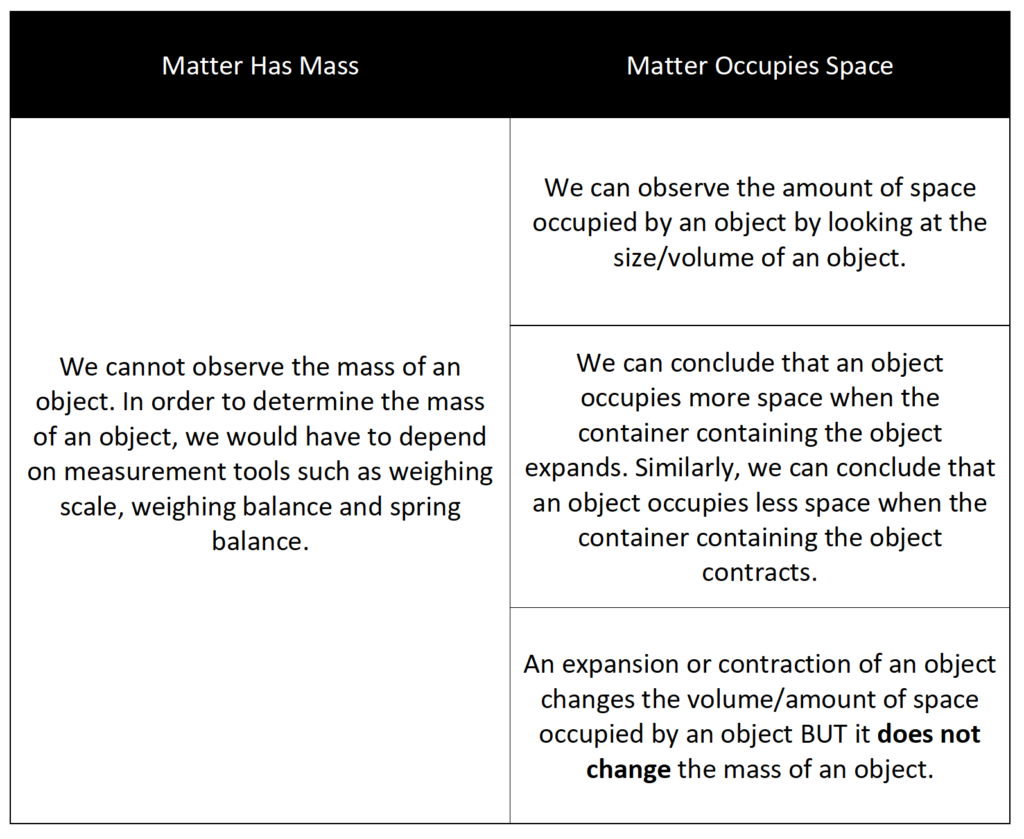In the previous article on Matter, we discussed the key properties of matter:
• Matter has mass
• Matter occupies space

Students generally do not have difficulties in understanding these properties of matter.  Instead, it is the application of these properties that students typically struggle with.

Hence, in this article, we will be discussing the application of the matter concepts we have learned in our earlier post.

## Application QuestionJohn placed a balloon in a beaker of hot water. He recorded the change in the size of the balloon as shown below.

Relating to the properties of matter, what can you observe about the balloon?

## Here’s The Thought Process

One keyword that I often see being used in examination questions is the word “observe” – What can you observe from the diagram below?

What does this keyword “observe” exactly mean? Using the simplest interpretation of this word, to “observe” simply means to “see”. In other words, the question can be phrased as: “What can you see from the diagram below?”.

In the topic of Matter, the basic properties of matter are:

1. Matter has mass
2. Matter occupies space

Relating back to the concepts you have learned about matter, you have to ask yourself 2 important questions:

1. Can we “see” an increase or a decrease in mass?
1. The answer is NO! We cannot see mass. In order to determine the mass of an object, we have to rely on a weighing scale/spring balance.
2. Can we “see” an increase or a decrease in the amount of space occupied?
1. The answer is YES! We can do that by looking at the change in the size of the object. When the size of the object increases, the object is occupying more space (expansion). When the size of the object decreases, the object is occupying less space (contraction).

In this example, we can see that the size/volume of the balloon increases. This shows that the air in the balloon is occupying more space.

However, it is important to note that the number of air particles in the balloon remains the same, as there was no air entering or escaping from the balloon. As such, we can state that the mass of the balloon does not change.

## Let Us Summarise And Recap What We Have Just LearnedIf you like our methodology, please click here to explore our Matter Techniques™ Masterclass for P5 & P6 students.